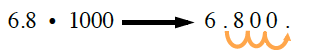Home > CC1MN > Chapter cc15 > Lesson cc15.1.3 > Problem5-26

5-26.

Multiply each pair of numbers below. Homework Help ✎

1. $68·100$

• 6800

1. $0.68·100$

1. $6.8·1000$

6800

1. Describe in words what is happening to the decimal point in each problem, (a) through (c) above.

• Example (c):
What happens to the decimal point?